# Samacheer Kalvi 8th Maths Book Solutions Term 2 Chapter 3 | Geometry Answers For Tamil Nadu Board

Samacheer Kalvi 8th Maths Book Solutions Term 2 Chapter 3 – Geometry is available here. The Samacheer Kalvi 8th Maths book answers of Term 2 Chapter 3, available at BYJU’S, contain step by step explanations designed by our Mathematics experts. All these important questions are based on the new pattern prescribed by the Tamil Nadu board. Students can also get the solutions of other chapters on Samacheer Kalvi 8th  Maths solutions. Students are advised to practise these Samacheer Kalvi Class 8 Maths Book Term 2 Chapter 3 questions so that they get used to solving different questions of various difficulty levels.

Term 2 Chapter 3 of the Samacheer Kalvi 8th Maths guide will help the students to solve problems related to the Pythagorean theorem and its converse, Hypotenuse theorem, construction of trapeziums, construction of parallelograms.

### Samacheer Kalvi 8th Maths Term 2 Chapter 3: Geometry Book Exercise 3.1 Questions and Solutions

Question 1: Check whether given sides are the sides of right-angled triangles, using Pythagoras theorem: 8,15,17.

Solution:

8, 15, 17

a = 8, b = 15, c = 17

a2 + b2 = 82 + 152

= 64 + 225

= 289

c2 = 172 = 289

a2 + b2 = c2

The given measure of the sides of the triangle forms a right angled triangle.

Question 2: An isosceles triangle has equal sides each 13cm and a base 24cm in length. Find its height.

Solution:

In an isosceles triangle, the altitude divides the base into two equal parts.

In the below figure, △ABC is an isosceles triangle with AD as its height.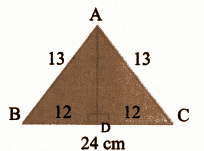In the figure, AD is the altitude and △ABD is a right triangle.

By Pythagoras theorem,

132 – 122 = 169 – 144 = 25

Question 3: Find the distance between the helicopter and the ship.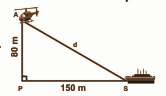Solution:

AS is the distance between the helicopter and the ship.

△APS is a right-angled triangle.

By Pythagoras theorem,

AS2 = AP2 + PS2

= 802 + 1502

= 6400 + 22500

= 28900

= 1702

AS = 170m

The distance between the ship and the helicopter is 170m.

### Samacheer Kalvi 8th Maths Term 2 Chapter 3: Geometry Book Exercise 3.2 Questions and Solutions

Question 1: Find the length of the support cable required to support the tower with the floor.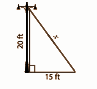Solution:

From the figure, by Pythagoras theorem,

x2 = 202 + 152

= 400 + 225

= 625

= 252

x = 25ft

The length of the support cable required to support the tower with the floor is 25ft.

Question 2: The diagonals of a rhombus are 12cm and 16cm. Find its perimeter.

Solution: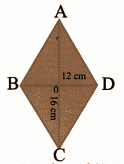AO = CO = 8cm

BO = DO = 6cm

The diagonals of the rhombus bisect each other at right angles.

In △AOB,

AB2 = AO2 + OB2

= 82 + 62

= 64 + 36

= 100

= 102

AB = 10cm

Since it is a rhombus, all the four sides are equal.

AB = BC = CD = DA

Perimeter = 10 + 10 + 10 + 10 = 40cm

Question 3: In the figure, find AR.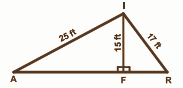Solution:

△AFI and △FRI are right triangles.

By Pythagoras theorem,

AF2 = AT2 – FT2

= 252 – 152

= 625 – 225

= 400

= 202

AF = 20 ft — (1)

FR2 = RI2 – FI2

= 172 – 152

= 289 – 225

= 64

= 82

FR = 8ft

AR = AF + FR = 20 + 8 = 28 ft

### Samacheer Kalvi 8th Maths Term 2 Chapter 3: Geometry Book Exercise 3.3 Questions and Solutions

Question 1: Construct the following trapeziums with the given measures and also find their area:

[i] AIMS with AI || SM, AI = 6cm, IM = 5cm, AM = 9cm and MS = 6.5cm.

[ii] BIKE with BI || EK, BI = 4cm, IK = 3.5cm, BK = 6cm and BE = 3.5cm.

[iii] CUTE with CD || ET, CU = 7cm, ∠UCE = 80° CE = 6cm and TE = 5cm.

Solution:

[i] AIMS with AI || SM, AI = 6cm, IM = 5cm, AM = 9cm and MS = 6.5cm.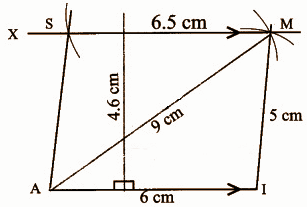Steps for construction:

1] A line segment AI = 6cm.

2] Draw two arcs of radii 9cm and 5cm with A and I as centres which cut at M.

3] Join AM and IM.

4] Draw MX parallel to AI.

5] An arc of radius 6.5cm is drawn with M as the centre which cuts at MX at S.

6] AIMS is the required quadrilateral.

Area of the trapezium AIMS = [1 / 2] * h * (a + b) sq.units

= [1 / 2] * (4.6) * (6 + 6.5)

= [1 / 2] * [4.6] * [12.5]

= 28.75 sq.cm.

[ii] BIKE with BI || EK, BI = 4cm, IK = 3.5cm, BK = 6cm and BE = 3.5cm.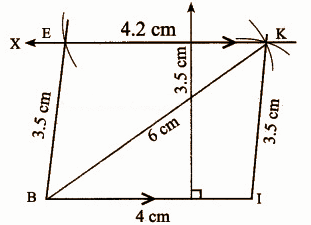Steps for construction:

1] A line segment BI = 4cm.

2] Draw two arcs of radii 6cm and 3.5cm with B and I as centres which cut at K.

3] Join BK and IK.

4] Draw KX parallel to BI.

5] An arc of radius 3.5cm is drawn with B as the centre which cuts at KX at E.

6] BIKE is the required trapezium.

Area of the trapezium BIKE = [1 / 2] * h * (a + b) sq.units

= [1 / 2] * (3.5) * (4 + 4.2)

= [1 / 2] * [3.5] * [8.2]

= 14.35 sq.cm.

[iii] CUTE with CD || ET, CU = 7cm, ∠UCE = 80° CE = 6cm and TE = 5cm.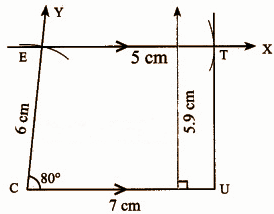Steps for construction:

1] A line segment CU = 7cm.

2] Construct an angle ∠UCE = 80° at C.

3] Draw an arc of radius 6cm with C as the centre which cuts CY at E.

4] Draw EX parallel to CU.

5] An arc of radius 5cm is drawn with E as the centre which cuts at EX at T.

6] CUTE is the required trapezium.

Area of the trapezium CUTE = [1 / 2] * h * (a + b) sq.units

= [1 / 2] * (5.9) * (7 + 5)

= [1 / 2] * [5.9] * 

= 35.4 sq.cm.

### Samacheer Kalvi 8th Maths Term 2 Chapter 3: Geometry Book Exercise 3.4 Questions and Solutions

Question 1: Construct the following parallelograms with the given measurements and find their area.

a. ARTS, AR = 6cm, RT = 5cm and ∠ART = 70°.

b. BANK, BA = 7cm, BK = 5.6cm and ∠KBA = 85°.

c. CAMP, CA = 6cm, AP = 8cm and CP = 5.5cm.

Solution:

a. ARTS, AR = 6cm, RT = 5cm and ∠ART = 70°.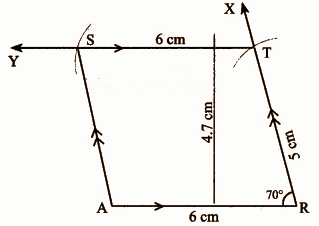Steps of construction:

1] A line segment AR = 6cm.

2] Construct an angle ∠ART = 70° at R on AR.

3] Draw an arc of radius 5cm with R as the centre which cuts RX at T.

4] Draw TY parallel to AR through T.

5] An arc of radius 6cm is drawn with T as the centre which cuts at TY at S. Join AS.

6] ARTS is the required parallelogram.

Area of the parallelogram ARTS = b * h sq.units

= 6 * (4.7)

= 28.2 sq.cm.

b. BANK, BA = 7cm, BK = 5.6cm and ∠KBA = 85°.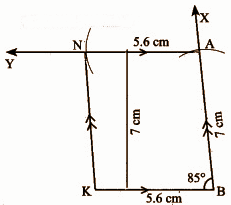Steps of construction:

1] A line segment KB = 5.6cm.

2] Construct an angle of ∠KBA = 85° at B.

3] Draw an arc of radius 7cm with B as the centre on BX.

4] Draw AY parallel to KB.

5] An arc of radius 5.6cm is drawn with A as the centre which cuts at AY at N. Join KN.

6] BANK is the required parallelogram.

Area of the parallelogram BANK = b * h sq.units

= 5.6 * (7)

= 39.2 sq.cm.

c. CAMP, CA = 6cm, AP = 8cm and CP = 5.5cm.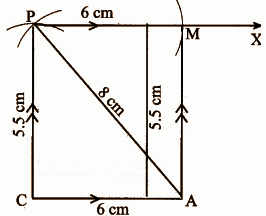Steps of construction:

1] A line segment CA = 6cm.

2] Draw an arc of length 5.5cm with C as the centre and with A as the centre, draw another arc of length 8cm.

3] Mark the intersecting point of both the arcs as P.

4] Draw a line PX parallel to CA.

5] An arc of radius 6cm is drawn with P as the centre which cuts at PX at M. Join AM.

6] CAMP is the required parallelogram.

Area of the parallelogram CAMP = b * h sq.units

= 6 * (5.5)

= 33 sq.cm.